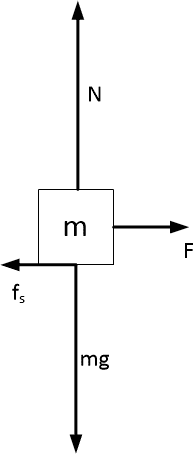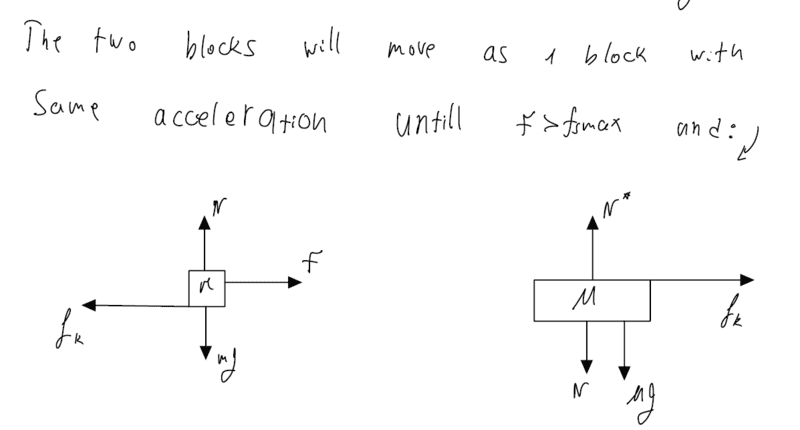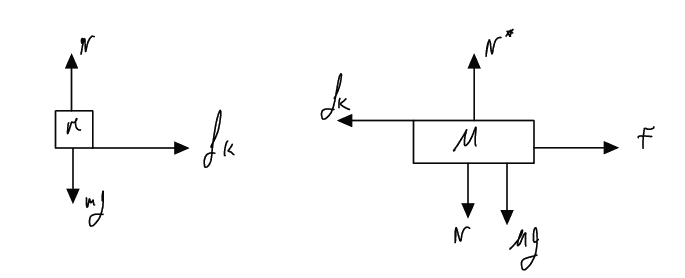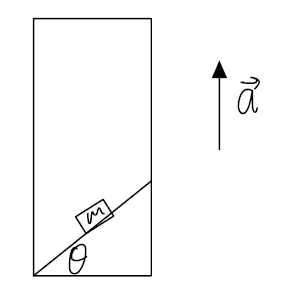# Newton's laws — Two stacked blocks sliding on a frictionless floor

nirlalush
Homework Statement:
block on block fricton , and inclined plane in elevator
Relevant Equations:
f=ma
hello , I am adding pdf with the questions and what i tried to do to solve them.
in the first question my teacher just taught us that if there is a frictionless floor and i push the upper block (m) with force F toward me then if the static friction will be toward me too. so the lower block M is going to go the opposite way of the force and i don't understand how it is logical. and if the m slide on M then its kinetic friction and the directions of the friction switch so the friction would be againt the F.
in short my question is if someone can give me a better explination about this specific problem.

in the second question i struggle to understand how to set the X and Y axis and the forces and what is Normal force if the elevator accelerates upward with a.

#### Attachments

• Question.pdf
397.9 KB · Views: 58

Homework Helper
Hello @nirlalush ,##\qquad## !​
i push the upper block (m) with force F toward me then if the static friction will be toward me too
friction is a force between objects, and according to Newton 3 the force on the one object is equal and opposed to the force on the other. So if you say 'toward me', you also have to state which of the two objects you are referring to...

so the lower block M is going to go the opposite way of the force and i don't understand how it is logical

And here I suspect a case of miscommunication ! You draw the friction force that block ##M## exerts on block ##m## in the same direction as the pulling force ##F##. That is indeed not logical !

You can approach this exercise by first thinking about a simpler case: You pull on block ##m## on a floor with friction (coefficient ##\mu_s##). (This is equivalent to letting the mass of block ##m## go to infinity -- such a thought experiment is ver often quite useful !). If you don't pull too hard (##F\lt f_{s,\ max} ##) the FBD is a well-balancedFriction force ##f_s## has a maximum value ## f_{s,\ max} = \mu N \ ## and as long as ##F## does not exceed that value, the block will stay in position.

Clear so far ? And does it match your intuition/experience ?

So the friction force on the upper block works to the left, not to the right.
This is also the case when the lower block is not infinitely heavy. Logical ?

So, in what direction is the friction force on the lower block ?

We will deal with the elevator after we have completed the block exercise.

##\ ##

•Delta2, nirlalush and berkeman
nirlalush
hello, thanks for the reply and i hope you understand me , english isn't my native language .
i uploaded another pdf with the way i would solve this. and what i understand is , if my solution is right , that my teacher was wrong and i need to ask him that again .

Edit:
hi, i uploaded another file and i wrote all cases i can think, of this block above block problems. i did a free body diagram , its very long but if you can or someone to see if i did it correct and if i understand what happens in each case.(if i do the free body diagram correctly than all question of this kind would be easy)
thank you very much for your help !

#### Attachments

• Question (1).pdf
538.8 KB · Views: 45
• Block on block scenarios .pdf
1.2 MB · Views: 51
Last edited:
Homework Helper
english isn't my native language
It isn't mine eithermy teacher was wrong
I still suspect a mistake in communication.

Right. So the friction force on the lower block works to the right; same direction as ##F##.
The free body diagrams raise a few questions:
One I can answer: Force ##N## is ##mg## and ##N^*## is ##Mg + N##. But you really should distinguish more clearly between lower case ##m## and upper case ##M##.
The other question is about ##f_k## which you make appear greater than ##F##. Why ? Under what conditions would that be the case ?

The problem statement asks for the minimum ##F## required to start the block ##m## to slide on block ##M##.

I agree with with your statement " ##\ f_{s,max} = \mu mg\ ##. "

I do not agree with your statement "The two blocks will move as 1 block with the same acceleration -- until ##\ F\gt f_{s,max}\ ##. " It seems to me you don't either, because you derive (3) ##\ F_M = \mu mg(1+{m\over M})##, (so more than ##f_{s,max}\ ##).

And I think your ##F_M## is the correct answer. I would be surprised if teacher disagrees.

##\ ##

•nirlalush
nirlalush
hello , thanks for the help.
when i wrote "the two blocks wIl move as 1 " and you showed me that the solution i found refutes this claim,
i have a question about how it could be that F would be equal to fsmax and that doesn't cause the little case m to change it's state to kinetic friction. because if it indeed was above infinity mass then it would change.

and when did i wrote fk bigger then F?

Last edited:
Homework Helper
when i wrote "the two blocks wIl move as 1 " and you showed me that the solution i found refutes this claim
Ah, that was formulated a bit awkward. What I meant was: I agree that the two blocks move as 1 with the same acceleration until ##F \gt f_{s,max}\ ##, but even a bit further than until ##F= f_{s,max}\ \ ## -- as you show. Namely until ##F = f_{s,max} (1+{m\over M})##and when i put fk bigger then F?

In the picture ##f_k## looks a bit bigger than ##F##. That can never be the case: if you are pulling to the right there is no way the upper block can accelerate to the left.

I suppose you use the symbol ##f_k## for the kinetic friction force. But in the problem statement I only find one ##\mu##, so the best that can be done is to assume ##\mu_k = \mu_s = \mu##.

All this refers to Question (1).pdf.

--------

I see you added picture "Block on block scenarios.pdf" . Six pages !

Option 1: There force F is acting on the lower block ##M##. Is this a new exercise, or is this the exercise as it was originally meant by teacher ? Because this is a completely different exercise than the one in Question.pdf and Question (1).pdf.

If this is the actual exercise, I suspect that's where the source of miscommunication is hiding !

I certainly do not agree withas the maximum friction force between ##m## and ##M##.

And it is unclear to me what is going on on page 3 and in option 2. Where does this all come from ?

Could you learn a bit of ##\LaTeX## so we can eliminate a whole lot of miscommunication, confusion and duplications ? There is a button on the lower left:##\ ##

•nirlalush
nirlalush
\begin{aligned}\sum F_{my}=0\\ N-my=0\\ N=mg\\ f_{s}=\mu_{s} N=\mu _{s}mg\end{aligned}

that on the upper block m.

so in the x Axis of M
\begin{aligned}\sum F_{Mx}=Ma\\ F _{min}-f_{s\max}=M_{a}\\ F_{min}=\mu_{s}mg+M\mu_{s}g\\ F_{min}=\mu_{s}g\left(m+M\right) \end{aligned}

is it correct?

Homework Helper
Depends on what you are referring to. Is this the exercise from post #1 where you are pulling the upper mass ? I guess not.

Is this Option 1 in "Block on block scenarios.pdf" ? Where you are pulling the lower mass ##M\ ##, with free body diagramsthen: yes, I think that is right.

##\ ##

•nirlalush
nirlalush
thanks a lot man, i think i get the idea of this problems.
can you give me direction on how to put the x and y-axis on the second problem with the elevator ?

Homework Helper
Second problem:
We have a block with mass ##m## on an inclined plane (angle ##\theta## wrt horizontal) inside an elevator that is accelerating upward with accelration ##a##. What is the acceleration of block ##m## ?Is this with or without friction ?

In your free body diagram I am missing ##mg## (but you do show the components along and perpendicular to the inclined plane)

Can you do the exercise for ##a=0## ?
Or for ##\theta = 0##?

##\ ##

•nirlalush
nirlalush
ΣFy=ma
N-mg=ma
n=m(g+a)
that is the equation when the plane is with angle =0.

between the block and the plance the is not friction.

Homework Helper
Gold Member
2022 Award
ΣFy=ma
N-mg=ma
n=m(g+a)
that is the equation when the plane is with angle =0.

between the block and the plance the is not friction.
I haven't been following this thread. Is this question 2?

•nirlalush
nirlalush
i stuggle with the question of the inclined plane in accelerating elevator. he told me what i would do if the angle was 0 .
i saw in some threads that you need to put another F equals to ma even though the object doesn't feel that F force but i don't understand that.

Homework Helper
Gold Member
2022 Award
i saw in some threads that you need to put another F equals to ma
Then I would guess their approach is to use the reference frame of the elevator. In that frame, the elevator is not accelerating, and neither is anyone standing in it. In accelerating reference frames you have to introduce "fictitious" forces , like centrifugal force, to make the equations of motion work. To explain the additional normal force experienced by a passenger mass m you would add a force ma in the opposite direction to the reference frame's acceleration.

•nirlalush
nirlalush
can you tell me if there is another way? because i never solved problems with that technique and i don't understand why to add a force in the opposite way if the eleveator accelerates upward.

edit: you say that if I am in an elevator and it starts to accelerate then by Newton first law my body ,because there isn't a force exert on him, would want to stay put but the elevator accelerates upward and I am in it so i feel a "force" that push me down.
and if i add that fictious foce that means i treat the system as moving at constant speed ?

Last edited:
Homework Helper
Gold Member
2022 Award
can you tell me if there is another way? because i never solved problems with that technique and i don't understand why to add a force in the opposite way if the eleveator accelerates upward.

edit: you say that if I am in an elevator and it starts to accelerate then by Newton first law my body ,because there isn't a force exert on him, would want to stay put but the elevator accelerates upward and I am in it so i feel a "force" that push me down.
and if i add that fictious foce that means i treat the system as moving at constant speed ?
I am saying that if you choose yourself as your reference frame then, by definition, you are not accelerating. Yet, if the elevator is accelerating up, you feel an increased normal force from the floor. You interpret your acceleration as a force pushing you down. Indeed, if we accept such a force, ma, as one contributing to your motion then the net force acting on you is N-mg-ma up, where N is the normal force from the floor. Since N=m(g+a), the net force is zero and the acceleration that results is therefore zero, as required.

But using such a reference frame is optional. If you are more comfortable with inertial frames stick with those. In such a frame, you are accelerating up, as given by ma=N-mg. The equation is the same.

•nirlalush
nirlalush
I am saying that if you choose yourself as your reference frame then, by definition, you are not accelerating.
i'm sorry but it's hard to understand that, thanks for the help.
i didn't understand that sentence . you mean "you are not accelerating" but i do feel that forces are exert upon me then of course i feel that i accelerate ? no?

but in inertia frame i have acceleration up and the body slides on the plane so i don't understand how to set the x and y-axis and what equations to write.

Last edited:
Homework Helper
Gold Member
2022 Award
i'm sorry but it's hard to understand that, thanks for the help.
i didn't understand that sentence . you mean "you are not accelerating" but i do feel that forces are exert upon me then of course i feel that i accelerate ? no?

but in inertia frame i have acceleration up and the body slides on the plane so i don't understand how to set the x and y-axis and what equations to write.
When you stand still you can feel the normal force from the floor and the weight exerted on the lower parts of your body by the upper parts. Are you accelerating? In your 'frame of reference' you measure all motion relative to yourself. That is what a frame of reference means. Clearly, you are not moving relative to yourself. In your frame, you are stationary.
If you are accelerating upward in an elevator, this feels just the same as increased gravity. So, if you consider that you can feel the acceleration then, you would have to say that even when the elevator is stationary you feel an upward acceleration of g.

•nirlalush